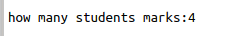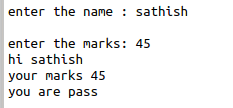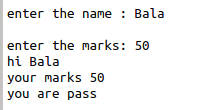• #5, First Floor, 4th Street Dr. Subbarayan Nagar Kodambakkam, Chennai-600 024 Landmark : Samiyar Madam
• pro@slogix.in
• +91- 81240 01111

### How to create class and object in python?

###### Description

To see how to create a class and object in python3.

###### Class

A class is simply a blueprint of a data that defines the characteristics and behavior of its data members.

Python starts with the keyword class followed by the name of the class and colon(:)

Eg.class student():

The body of the class which contains variables, data, and functions.

###### Object

Object is memory reference of a class.

Used to store data and functions defined inside a class.

Using object we can call the methods that are defined in the class.

Object should be within the class not with in the methods

###### Sample Code

#class classname(student)
class Student:
#method1
def __init__ (self,n , m =0 ):
self.name = n
self.marks = m
#method2
def display(self):
print(“hi”, self.name)
#method3
def calculate(self):
if (self.marks >= 75):
print(“you got first class”)

elif (self.marks >= 45):
print(“you are pass”)
else:
print(“you are fail”)
#get input from the user
n = int(input(“how many students marks:” ))

#initialize the loop for many students
i = 0
while (i < n):
name = input(“enter the name : “)
marks = int(input(“enter the marks: “))

#object creation for student class
s1=Student(name, marks)

#method calling statements
s1.display()
s1.calculate()
i=i+1
print(“************”)

###### Screenshots